BIOGRAPHY

M A R I A   V E R O N I C A

M A R I A   V E R O N I C A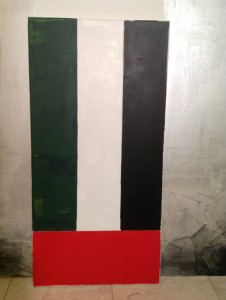P  E  R  F  O  R  M  A  N  C  E

P  E  R  F  O  R  M  A  N  C  E

T     o     d     a     y . . .
P  E  R  F  O  R  M  A  N  C  E

P  E  R  F  O  R  M  A  N  C  E

M A R I A  V E R O N I C A   L I V E S   A N D   W O R K S   C U R R E N T L Y   I N   D U B A I
P  E  R  F  O  R  M  A  N  C  E
P  E  R  F  O  R  M  A  N  C  E
U  N  I  T  E  D    A  R  A  B    E  M  I  R  A  T  E  S

P  E  R  F  O  R  M  A  N  C  E

P  E  R  F  O  R  M  A  N  C  E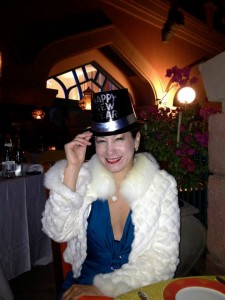P  E  R  F  O  R  M  A  N  C  E

P  E  R  F  O  R  M  A  N  C  E

She has been represented by Cube Gallery Arts, DIFC (Dubai International Financial Center)

P  E  R  F  O  R  M  A  N  C  E

P  E  R  F  O  R  M  A  N  C  E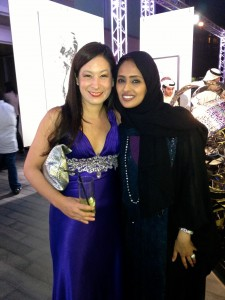P  E  R  F  O  R  M  A  N  C  E

Safa Al Hamed, Cube Arts and The Empty Quarter Gallery owner in Dubai

P  E  R  F  O  R  M  A  N  C  E

P  E  R  F  O  R  M  A  N  C  E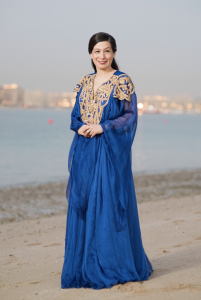P  E  R  F  O  R  M  A  N  C  E

M  A  R  I  A     V  E  R  O  N  I  C  A
P  E  R  F  O  R  M  A  N  C  E
V i s u a l   A r t i s t
G u a y a q u i l - P a r i s - D u b a i
P  E  R  F  O  R  M  A  N  C  E
P  E  R  F  O  R  M  A  N  C  E

From her early years María Verónica has shown her extraordinary talent in drawing, painting, portraiture and self-portraiture. She made portraits of prominent figures like Bjork, Isabelle Adjani, Pina Bausch, Dominique Mercy, Julie Stanzak, Helena Pikon, Placido Domingo, Bill Clinton, HH Sheikh Mohammed bin Rashid Al Maktoum, HH Sheikha Moza bint Nasser, Dr Hani and Nancy Sakla and diverse Ecuadorian, Latin American, European and Middle Eastern personalities.

In 2014 she set up in Dubai where she works now and became the first artist from Latin America represented by a gallery from the Emirates, Cube Arts Gallery, Dubai 2014, her first solo show in this gallery was the first one of an artist from Latin America in the Emirates. Today as a multidisciplinary artist she works with a variety of techniques: drawing, painting, printmaking, video art, video installation, video-poetry, digital books, performance and digital photography. Her life and avant-garde work make her a leading figure in the international contemporary art scene.

In Ecuador she worked with “La Galeria” in Quito (1997) and Madeleine Hollaender in Guayaquil (1995-1997). In 1996 Guayasamin invited her to collaborate with him in murals paintings for “La Capilla del Hombre” in Quito. She attended the School of Fine Arts, Central University in Quito, Painting and Printing specialization, where she was also Dean Assistant of Printing Department.
P  E  R  F  O  R  M  A  N  C  E

P  E  R  F  O  R  M  A  N  C  E

P  E  R  F  O  R  M  A  N  C  E

Y   e    s    t    e    r    d    a    y   .  .  .

P  E  R  F  O  R  M  A  N  C  E

P  E  R  F  O  R  M  A  N  C  E

1  9  9  1   -   1  9  9  6
P  E  R  F  O  R  M  A  N  C  E
School of Fine Arts, Central University in Quito, Ecuador, specialization Painting and Printing

P  E  R  F  O  R  M  A  N  C  E

1  9  9  4   -   1  9  9  6
P  E  R  F  O  R  M  A  N  C  E
Dean Assistant of Printing Department at School of Fine Arts, Quito Central University, Ecuador
g'lkdjr'lgdjf'lhjf'tlmh'lgnh'lf/tmnh'ol;gl/mdsf'/l.hnfkldbglkdrng;lh.fthvhfjasgf;dguydfuwydvjhwbdisahpocxjndkjbvjfbjlgmb;,.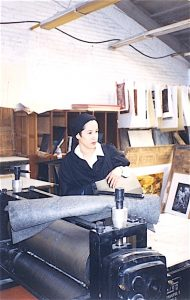P  E  R  F  O  R  M  A  N  C  E

P  E  R  F  O  R  M  A  N  C  E

P  E  R  F  O  R  M  A  N  C  E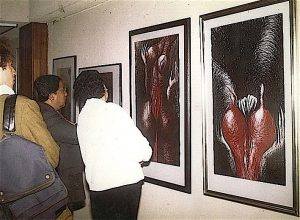P  E  R  F  O  R  M  A  N  C  E

P  E  R  F  O  R  M  A  N  C  E

P  E  R  F  O  R  M  A  N  C  E

P  E  R  F  O  R  M  A  N  C  E

1  9  9  6   -   1  9  9  7
P  E  R  F  O  R  M  A  N  C  E
Maria Veronica works with Guayasamin in Mural painting for "La Capilla del Hombre", Quito

P  E  R  F  O  R  M  A  N  C  E

P  E  R  F  O  R  M  A  N  C  E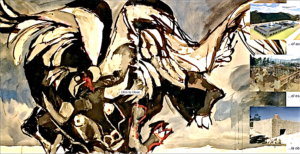P  E  R  F  O  R  M  A  N  C  E

P  E  R  F  O  R  M  A  N  C  E

P  E  R  F  O  R  M  A  N  C  E

P  E  R  F  O  R  M  A  N  C  E

1  9  9  7
P  E  R  F  O  R  M  A  N  C  E
She works with “La Galeria” in Quito and Madeleine Hollaender Gallery in Guayaquil, Ecuador

P  E  R  F  O  R  M  A  N  C  E

P  E  R  F  O  R  M  A  N  C  E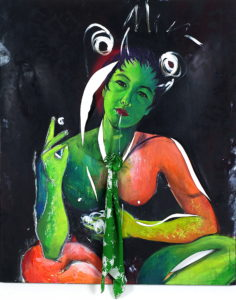P  E  R  F  O  R  M  A  N  C  E

P  E  R  F  O  R  M  A  N  C  E

P  E  R  F  O  R  M  A  N  C  E

P  E  R  F  O  R  M  A  N  C  E

P  E  R  F  O  R  M  A  N  C  E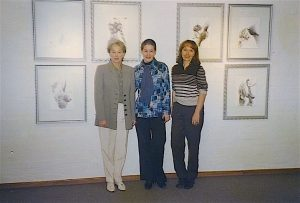P  E  R  F  O  R  M  A  N  C  E

La Galeria Quito 1998
Galerists Betty Wappenstein and Maria Elena Machuca

P  E  R  F  O  R  M  A  N  C  E

P  E  R  F  O  R  M  A  N  C  E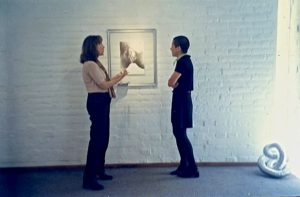P  E  R  F  O  R  M  A  N  C  E

Galerist Maria Elena Machuca

P  E  R  F  O  R  M  A  N  C  E

P  E  R  F  O  R  M  A  N  C  E

P  E  R  F  O  R  M  A  N  C  E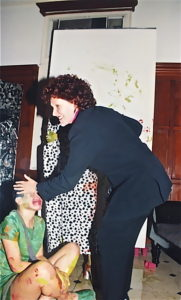P  E  R  F  O  R  M  A  N  C  E

P  E  R  F  O  R  M  A  N  C  E

P  E  R  F  O  R  M  A  N  C  E

P  E  R  F  O  R  M  A  N  C  E

P  E  R  F  O  R  M  A  N  C  E

1  9  9  8   -   2  0  1  3
P  E  R  F  O  R  M  A  N  C  E
She moved to Paris, where she lives and works as a multidisciplinary artist in different medias:
Drawing, Painting, Portrait, Art Video, Video Poetry, Multimedia Installation, Performance
and Photography

P  E  R  F  O  R  M  A  N  C  E

P  E  R  F  O  R  M  A  N  C  E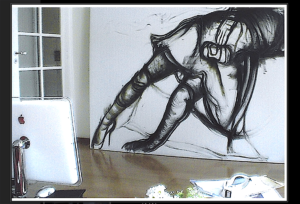P  E  R  F  O  R  M  A  N  C  E

P  E  R  F  O  R  M  A  N  C  E

P  E  R  F  O  R  M  A  N  C  E

P  E  R  F  O  R  M  A  N  C  E

P  E  R  F  O  R  M  A  N  C  E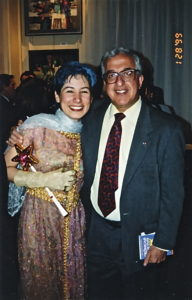P  E  R  F  O  R  M  A  N  C  E

Ambassador of Ecuador in Paris, Juan Cueva Jaramillo  1 9 9 8

P  E  R  F  O  R  M  A  N  C  E

P  E  R  F  O  R  M  A  N  C  E

P  E  R  F  O  R  M  A  N  C  E

P  E  R  F  O  R  M  A  N  C  E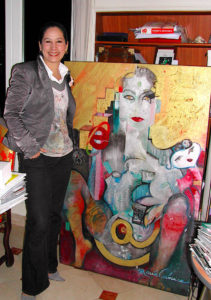P  E  R  F  O  R  M  A  N  C  E

Internet Goddess, Oil on Canvas, Paris 2oo1

P  E  R  F  O  R  M  A  N  C  E

P  E  R  F  O  R  M  A  N  C  E

P  E  R  F  O  R  M  A  N  C  E

P  E  R  F  O  R  M  A  N  C  E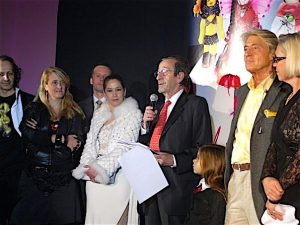P  E  R  F  O  R  M  A  N  C  E

Artists, Members of Unicef France and Commissaire Prisseur Pierre Cornette de Saint Cyr, Petit Palais Paris 2oo8

P  E  R  F  O  R  M  A  N  C  E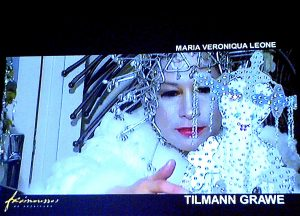P  E  R  F  O  R  M  A  N  C  E

P  E  R  F  O  R  M  A  N  C  E

P  E  R  F  O  R  M  A  N  C  E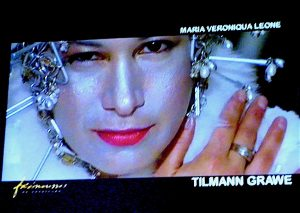P  E  R  F  O  R  M  A  N  C  E

P  E  R  F  O  R  M  A  N  C  E

P  E  R  F  O  R  M  A  N  C  E

[FG NO[ [FOXKG N[OKCG N[OKCG NO[KCG [OKC FNOK[CNGDORNG[ODKFNBO[KXDFNBOKXNFGBOK[
2  0  1  4   -   2  0  1  8

In 2O14 Maria Veronica set up in Dubai, UAE
where she has been represented by Cube Gallery Arts, DIFC (Dubai International Financial Center)

P  E  R  F  O  R  M  A  N  C  E

P  E  R  F  O  R  M  A  N  C  E

P  E  R  F  O  R  M  A  N  C  E

P  E  R  F  O  R  M  A  N  C  E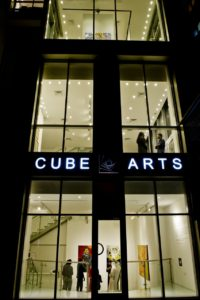P  E  R  F  O  R  M  A  N  C  E

P  E  R  F  O  R  M  A  N  C  E

P  E  R  F  O  R  M  A  N  C  E

P  E  R  F  O  R  M  A  N  C  E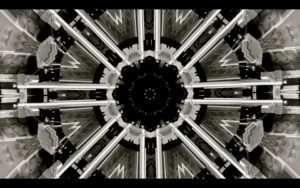P  E  R  F  O  R  M  A  N  C  E

P  E  R  F  O  R  M  A  N  C  E

P  E  R  F  O  R  M  A  N  C  E